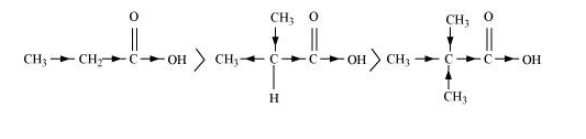# Explain the terms Inductive and Electromeric effects.Question:

Explain the terms Inductive and Electromeric effects. Which electron displacement effect explains the following correct orders of acidity of the carboxylic acids?

(a) $\mathrm{Cl}_{3} \mathrm{CCOOH}>\mathrm{Cl}_{2} \mathrm{CHCOOH}>\mathrm{ClCH}_{2} \mathrm{COOH}$

(b) $\mathrm{CH}_{3} \mathrm{CH}_{2} \mathrm{COOH}>\left(\mathrm{CH}_{3}\right)_{2} \mathrm{CHCOOH}>\left(\mathrm{CH}_{3}\right)_{3} \mathrm{C} \cdot \mathrm{COOH}$

Solution:

Inductive effect

The permanent displacement of sigma (σ) electrons along a saturated chain, whenever an electron withdrawing or electron donating group is present, is called inductive effect.

Inductive effect could be + I effect or – I effect. When an atom or group attracts electrons towards itself more strongly than hydrogen, it is said to possess – I effect. For example,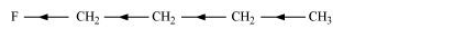When an atom or group attracts electrons towards itself less strongly than hydrogen, it is said to possess + I effect. For example,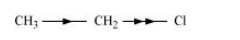Electrometric effect

It involves the complete transfer of the shared pair of $\pi$ electrons to either of the two atoms linked by multiple bonds in the presence of an attacking agent. For example,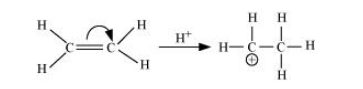Electrometric effect could be + E effect or – E effect.

+ E effect: When the electrons are transferred towards the attacking reagent

– E effect: When the electrons are transferred away from the attacking reagent

(a) $\mathrm{Cl}_{3} \mathrm{CCOOH}>\mathrm{Cl}_{2} \mathrm{CHCOOH}>\mathrm{ClCH}_{2} \mathrm{COOH}$

The order of acidity can be explained on the basis of Inductive effect ( $-I$ effect). As the number of chlorine atoms increases, the - I effect increases. With the increase in - I effect, the acid strength also increases accordingly.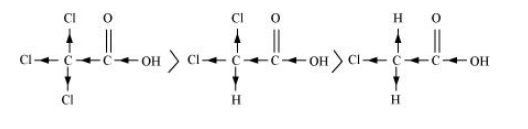(b) $\mathrm{CH}_{3} \mathrm{CH}_{2} \mathrm{COOH}>\left(\mathrm{CH}_{3}\right)_{2} \mathrm{CHCOOH}>\left(\mathrm{CH}_{3}\right)_{3}$ C.COOH

The order of acidity can be explained on the basis of inductive effect (+ I effect). As the number of alkyl groups increases, the $+I$ effect also increases. With the increase in $+$ I effect, the acid strength also increases accordingly.